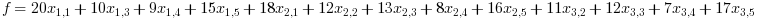# Assignment Problem

The public works department of a region has issued contracts for five new projects. Each of the three contractors who have shown interest in the projects has submitted bids for those projects. The bids given by contactors are as given below:

 Project 1 Project 2 Project 3 Project 4 Project 5 Contractor 1 20 - 10 9 15 Contractor 2 18 12 13 8 16 Contractor 3 - 11 12 7 17

The resource requirement of the contractors for each projects and the total resources available with them are as given below:

 Project 1 Project 2 Project 3 Project 4 Project 5 Available Resources Contractor 1 70 - 40 30 50 120 Contractor 2 65 45 40 35 55 100 Contractor 3 - 45 35 40 50 70

The objective of the contractors is to minimize the total cost.

Mathematical Formulation:

Minimize:Subjected to:

Resource Constraint:Each project can be completed by only one contractor:```clc; nProjects = 5; nContractors = 3; bids = [20 10000 10 9 15;18 12 13 8 16; 10000 11 12 7 17]; reqResource = [70 10000 40 30 50;65 40 40 35 55; 10000 45 35 40 50]; availResource = [120; 100; 70]; nVar = nProjects*nContractors; // Calculated the dimension of the problem IntCon = 1:nVar; // Indicating the integer variables lb = zeros(1,nVar); ub = ones(1,nVar); // Linear constraints // Linear equality constraints beq = ones(nProjects,1); for i = 1:nProjects Aeq(i,i:nProjects:nVar) = 1; end // Linear inequality constraints b = availResource; for j = 1:nContractors index = (j-1)*nProjects+1:j*nProjects; A(j,index) = reqResource(j,:); end // Objective function for j = 1:nContractors index = (j-1)*nProjects+1:j*nProjects; Cost(index,1) = bids(j,:)'; end options = list("time_limit", 2500); [xopt,fopt,status,output] = symphonymat(Cost,IntCon,A,b,Aeq,beq,lb,ub,options); // Result representation disp(output) for j = 1:nContractors index = (j-1)*nProjects+1:j*nProjects; Contractor(j).Project = string(find(xopt(index)==1)); end ResolurceUtilized = ((A*xopt)./b)*100; for j = 1:nContractors disp(strcat(["Projects assigned to contractor",string(j)," : ", Contractor(j).Project],' ')); disp(strcat(["Resource utilized by contractor ",string(j)," : ",string(ResolurceUtilized(j)),"%"])); end disp(strcat(["Total cost : ",string(fopt)]))```

Expected Output:

 ```Note: Time limit has been set to 2500.00. An optimal solution has been found. Iterations: 1 Projects assigned to contractor 1 : 3 5 Resource utilized by contractor 1 : 75% Projects assigned to contractor 2 : 1 4 Resource utilized by contractor 2 : 100% Projects assigned to contractor 3 : 2 Resource utilized by contractor 3 : 64.286% Total cost : 62```